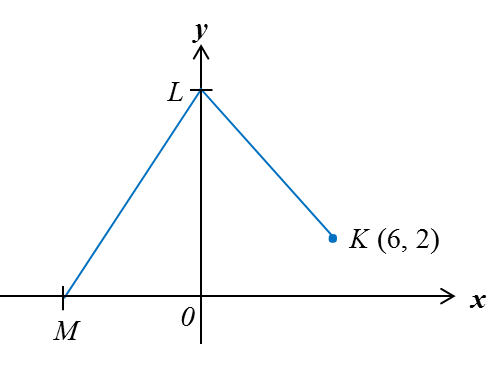# 10.4.2 Gradient of a Straight Line, PT3 Focus Practice

Question 6:
Diagram below shows a straight line RS with equation 3y = –px – 12, where p is a constant.It is given that OR: OS = 3 : 2.
Find the value of p.

Solution:
Method 1:
Substitute x= –6 and y = 0 into 3y = –px – 12:
3(0) = –p (–6) – 12
0 = 6p – 12
–6p = –12
p = 2

Method 2:
OR: OS = 3 : 2
$\begin{array}{l}\frac{OR}{OS}=\frac{3}{2}\\ \frac{6}{OS}=\frac{3}{2}\\ OS=6×\frac{2}{3}=4\text{units}\end{array}$

Coordinates of = (0, –4)
Gradient of the straight line RS = $-\frac{-4}{-6}=-\frac{2}{3}$

Given 3y = –px – 12
Rearrange the equation in the form y = mx + c
$\begin{array}{l}y=-\frac{p}{3}x-4\\ \text{Gradient of the straight line}RS=-\frac{P}{3}\\ \therefore -\frac{P}{3}=-\frac{2}{3}\\ \text{}P=2\end{array}$

Question 7:The above diagram shows two straight lines, KL and LM, on a Cartesian plane. The distance KL is 10 units and the gradient of LM is 2. Find the x-intercept of LM.

Solution:Let point be = (0, 2).
Using Pythagoras’ Theorem,
LN = √102 – 62 = 8
Point L = (0, 2 + 8) = (0, 10)
y-intercept of LM = 10

$\begin{array}{l}\text{Using the gradient formula,}m=-\frac{\text{y-intercept}}{\text{x-intercept}}\\ 2=-\left(\frac{10}{\text{x-intercept}}\right)\\ \text{x-intercept of}LM=-\frac{10}{2}=-5\end{array}$